# C# For Dummies

## Chapter 11. Creating and Using Objects

Write a program, which reads from the console a year and checks if it is a leap year.

Guidelines: Use DateTime.IsLeapYear(year).

Solution:
static void Main(string[] args)
{
Console.Write("Enter year: ");
if ((((year % 4) == 0) && ((year % 100) != 0) || ((year % 400) == 0)))
Console.WriteLine("{0} is leap year.", year);
else
Console.WriteLine("{0} isn't a leap year.", year);
}

2. Write a program, which generates and prints on the console 10 random numbers in the range [100, 200].

Guidelines: Use the class Random. You may generate random numbers in the range [100, 200] by calling Random.Next(100, 201).
Solution:
static void Main(string[] args)
{
Random r = new Random();
for (int i = 0; i < 10; i++)
Console.WriteLine(r.Next(100, 201));
}

3. Write a program, which prints, on the console which day of the week is today.

Guidelines: Use DateTime.Today.DayOfWeek.

Solution:
static void Main(string[] args)
{
Console.Write("Enter year: ");
Console.Write("Enter month: ");
Console.Write("Enter day: ");

DateTime dateValue = new DateTime(year, month, day);
Console.WriteLine(dateValue.ToString("ddd"));
}

4. Write a program, which prints on the standard output the count of days, hours, and minutes, which have passes since the computer is started until the moment of the program execution. For the implementation use the class `Environment`.

Guidelines: Use the property `Environment.TickCount`, in order to get the count of passed milliseconds. Use the fact that one second has 1,000 milliseconds; one minute has 60 seconds; one hour has 60 minutes and one day has 24 hours.

Solution: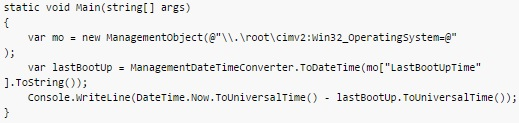5. Write a program which by given two sides finds the hypotenuse of a right triangle. Implement entering of the lengths of the sides from the standard input, and for the calculation of the hypotenuse use methods of the class `Math`.

Guidelines: The hypotenuse of a rectangular triangle could be found with the Pythagorean Theorem: `a2 + b2 = c2`, where `a` and `b` are the two sides, and `c` is the hypotenuse. Take square root of the two sides of the equation in order to get the length of the hypotenuse. Use the `Sqrt(…)` methods of the `Math` class.

Solution:
static void Main(string[] args)
{
Console.Write("First side: ");
Console.Write("Second side: ");

Console.Write("Hypotenuse is: " + Math.Sqrt(Math.Pow(a,2) + Math.Pow(b,2)));
}

Write a program which calculates the area of a triangle with the following given:
a. Three sides;
b. side and the altitude to it;
c. two sides and the angle between them in degrees.

Guidelines: For the first sub-problem of the task use the Heron’s Formula: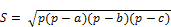, where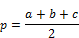.
For the second sub-problem use the formula: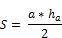.
For the third sub-problem use the formula: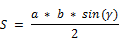Solution:
7. Define your own namespace `CreatingAndUsingObjects` and place in it two classes `Cat` and `Sequence`, which we used in the examples of the current chapter. Define one more namespace and make a class, which calls the classes `Cat` and `Sequence`, in it.

Guidelines: Make a new project in Visual Studio, right click on the folder and choose the menu Add → New Folder. Then enter the name of the folder and press [Enter], right click on the newly made folder and choose Add → New Item… from the list choose Class, for the name of the new class enter Cat and press [Add]. Change the definition of the newly created class with the definition, which we gave to this chapter, to put the classes in a namespace. Make the same to the class `Sequence`.

Solution:
8. Write a program which creates 10 objects of type Cat, gives them names `CatN`, where `N` is a unique serial number of the object, and in the end call the method `SayMiau()` for each of them. For the implementation use the namespace `CreatingAndUsingObjects`.

Guidelines: Create an array with 10 elements of type `Cat`. Create 10 objects of type Cat in a loop (use a constructor with parameters) and assign them to the corresponding element of the array. For the serial number of the objects use the method `NextValue()` of the `Sequence` class. In the end again in an array use the method `SayMiau()` for each of the array elements.

Solution:
9. Write a program, which calculates the count of workdays between the current date and another given date after the current (inclusive). Consider that workdays are all days from Monday to Friday, which are not public holidays, except when Saturday is a working day. The program should keep a list of predefined public holidays, as well as a list of predefined working Saturdays.

Guidelines: Use the class `System.DateTime` and the methods in it. You can execute a loop from the current date (`DateTime.Now.Date`) to the end date, consecutively incrementing the day by the method `AddDays(1)` and count the working days according to your country (e.g. all days except Saturday and Sunday and a few fixed non-working official holidays).

Solution:

10. You are given a sequence of positive integer numbers given as string of numbers separated by a space. Write a program, which calculates their sum. Example: "43 68 9 23 318" = 461.

Guidelines: Use `String.Split(' ')` to split the string by spaces. Then use `Int32.Parse(…)` to extract the separate numbers from the obtained string array as int values and sum them.

Solution:

11. Write a program, which generates a random advertising message for some product. The message has to consist of laudatory phrase, followed by a laudatory story, followed by author (first and last name) and city, which are selected from predefined lists. For example, let’s have the following lists:
- Laudatory phrases: {"The product is excellent.", "This is a great product.", "I use this product constantly.", "This is the best product from this category."}.
- Laudatory stories: {"Now I feel better.", "I managed to change.", "It made some miracle.", "I can’t believe it, but now I am feeling great.", "You should try it, too. I am very satisfied."}.
- First name of the author: {"Dayan", "Stella", "Hellen", "Kate"}.
- Last name of the author: {"Johnson", "Peterson", "Charls"}.
- Cities: {"London", "Paris", "Berlin", "New York", "Madrid"}.
Then the program would print randomly generated advertising message like the following:
 I use this product constantly. You should try it, too. I am very satisfied. -- Hellen Peterson, Berlin

Guidelines: Използвайте класа `System.Random` и неговия метод `Next(…)`.

Solution:
- real numbers, for example `5, 18.33, 3.14159, 12.6;`
- arithmetic operations: `+, -, *, /` (with their standard priorities);
- mathematical functions: `ln(x), sqrt(x), pow(x, y);`
Note that the numeral expressions have priorities, for example the expression `-1 + 2 + 3 * 4 - 0.5 = (-1) + 2 + (3 * 4) - 0.5 = 12.5.` Guidelines: Calculating a numeral expression is quite hard and is unlikely a beginner programmer to solve it correctly without external help. As a start check out the article in Wikipedia about the "Shunting-yard algorithm" describing how to convert an expression from to postfix notation (reversed Polish notation), and the article about calculating a postfix expression. There are really much special cases, so be sure to test your solution carefully.